• Slides: 25Ch. 2: Getting Started 1About this lecture • Study a few simple algorithms for sorting – Insertion Sort – Selection Sort (Exercise) – Merge Sort • Show why these algorithms are correct • Try to analyze the efficiency of these algorithms (how fast they run) 2The Sorting Problem Input: A list of n numbers Output: Arrange the numbers in increasing order Remark: Sorting has many applications. E. g. , if the list is already sorted, we can search a number in the list faster 3Insertion sort • A good algorithm for sorting a small number of elements • It works the way you might sort a hand of playing cards: – Start with an empty left hand the cards face down on the table – Then remove one card at a time from the table, and insert it into the correct position in the left hand – To find the correct position for a card, compare it with each of the cards already in the hand, from right to left – Finally, the cards held in the left hand are sorted 4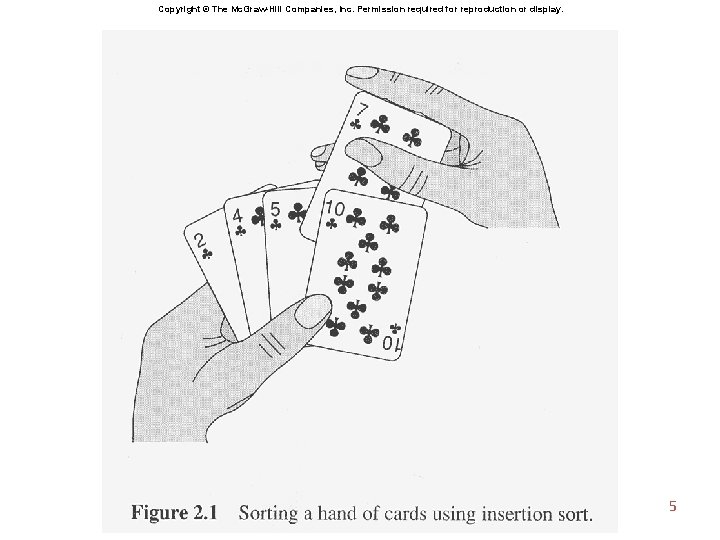Copyright © The Mc. Graw-Hill Companies, Inc. Permission required for reproduction or display. 5Insertion Sort • Operates in n rounds • At the kth round, Swap towards left side ; Stop until seeing an item with a smaller value. …… kth item Question: Why is this algorithm correct? 6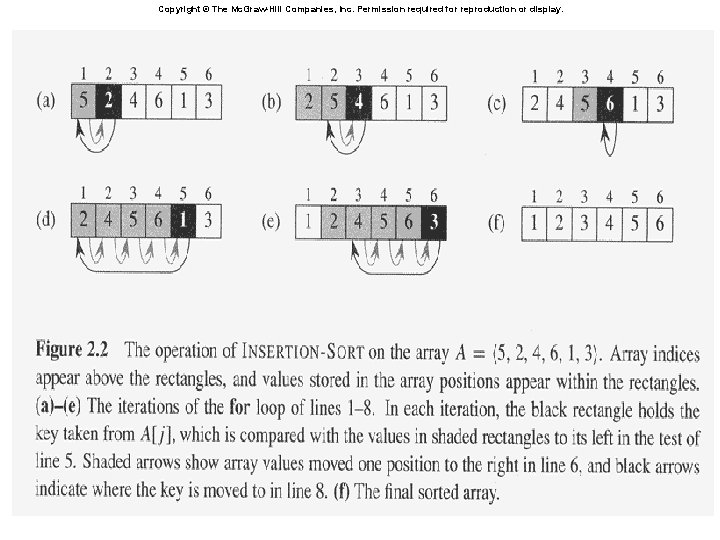Copyright © The Mc. Graw-Hill Companies, Inc. Permission required for reproduction or display. 7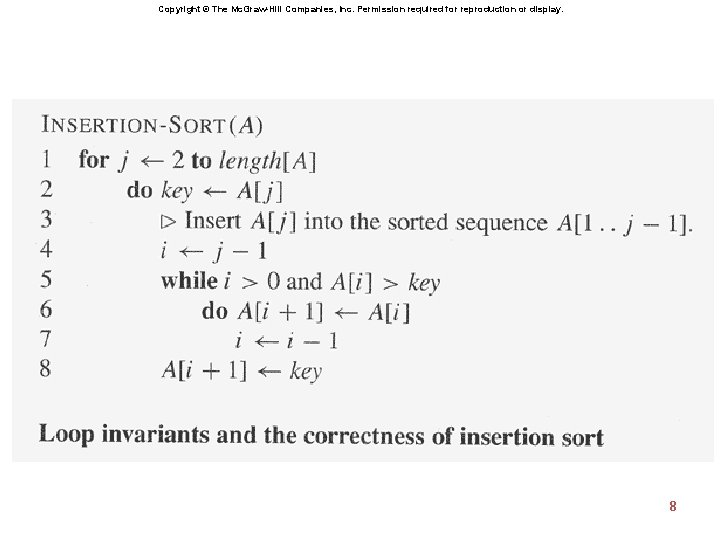Copyright © The Mc. Graw-Hill Companies, Inc. Permission required for reproduction or display. 8Correctness of Insertion Sort • Initialization: It is true prior to the first iteration of the loop • Maintenance: If it is true before an iteration of the loop, it remains true before the next iteration • Termination: When the loop terminates • Loop invariant: At the start of each iteration of the “outer” for loop— the loop indexed by j— the subarray A[1. . j-1] consists of the elements originally subarray A[1. . j-1] but in sorted order 9Divide and Conquer • Divide a big problem into smaller problems solve smaller problems separately combine the results to solve original one • This idea is called Divide-and-Conquer • Smart idea to solve complex problems (why? ) • Can we apply this idea for sorting ? 10Divide-and-Conquer for Sorting • What is a smaller problem ? E. g. , sorting fewer numbers Let’s divide the list to two shorter lists • Next, solve smaller problems (how? ) • Finally, combine the results “merging” two sorted lists into a single sorted list (how? ) 11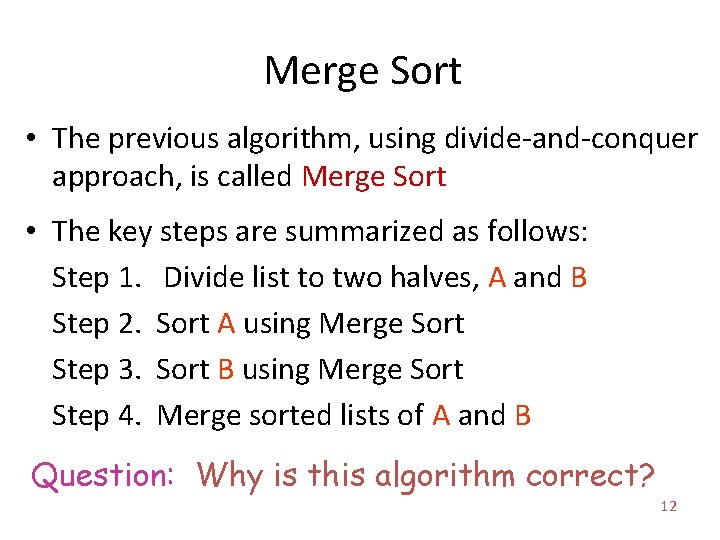Merge Sort • The previous algorithm, using divide-and-conquer approach, is called Merge Sort • The key steps are summarized as follows: Step 1. Divide list to two halves, A and B Step 2. Sort A using Merge Sort Step 3. Sort B using Merge Sort Step 4. Merge sorted lists of A and B Question: Why is this algorithm correct? 12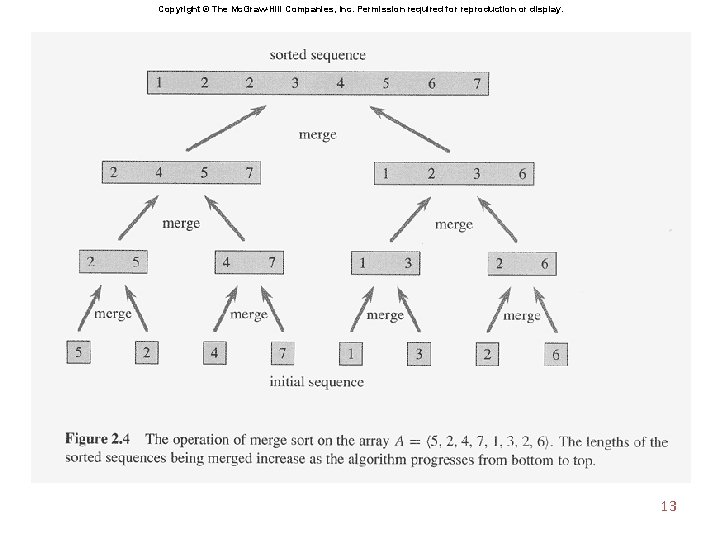Copyright © The Mc. Graw-Hill Companies, Inc. Permission required for reproduction or display. 13Analyzing the Running Times • Which of previous algorithms is the best? • Compare their running time on a computer – But there are many kinds of computers !!! Standard assumption: Our computer is a RAM (Random Access Machine), so that – each arithmetic (such as , , , ), memory access, and control (such as conditional jump, subroutine call, return) takes constant amount of time 14Analyzing the Running Times • Suppose that our algorithms are now described in terms of RAM operations we can count # of each operation used we can measure the running time ! • Running time is usually measured as a function of the input size – E. g. , n in our sorting problem 15Insertion Sort (Running Time) The following is a pseudo-code for Insertion Sort. Each line requires constant RAM operations. Why ? tj = # of times key is compared at round j 16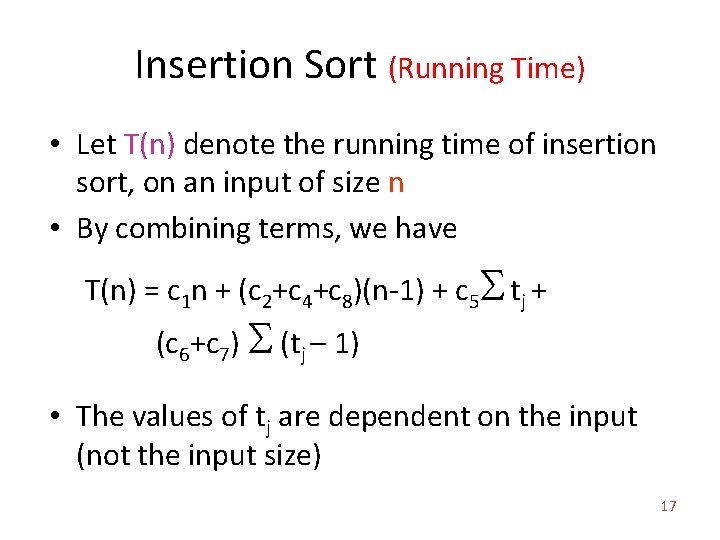Insertion Sort (Running Time) • Let T(n) denote the running time of insertion sort, on an input of size n • By combining terms, we have T(n) = c 1 n + (c 2+c 4+c 8)(n-1) + c 5 S tj + (c 6+c 7) S (tj – 1) • The values of tj are dependent on the input (not the input size) 17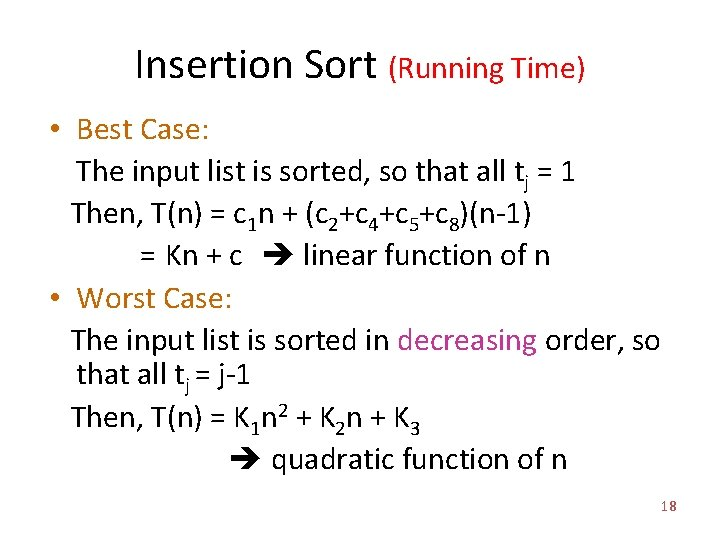Insertion Sort (Running Time) • Best Case: The input list is sorted, so that all tj = 1 Then, T(n) = c 1 n + (c 2+c 4+c 5+c 8)(n-1) = Kn + c linear function of n • Worst Case: The input list is sorted in decreasing order, so that all tj = j-1 Then, T(n) = K 1 n 2 + K 2 n + K 3 quadratic function of n 18Worst-Case Running Time • In our course (and in most CS research), we concentrate on worst-case time • Some reasons for this: 1. Gives an upper bound of running time 2. Worst case occurs fairly often • Remark: Some people also study average-case running time (they assume input is drawn randomly) 19Try this at home • Revisit pseudo-code for Insertion Sort – make sure you understand what’s going on • Write pseudo-code for Selection Sort 20Merge Sort (Running Time) The following is a partial pseudo-code for Merge Sort. The subroutine MERGE(A, p, q, r) is missing. Can you complete it? Hint: Create a temp array for merging 21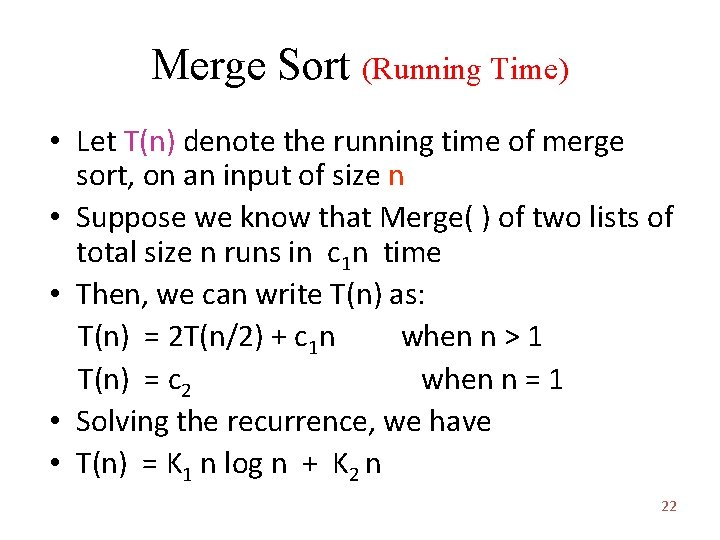Merge Sort (Running Time) • Let T(n) denote the running time of merge sort, on an input of size n • Suppose we know that Merge( ) of two lists of total size n runs in c 1 n time • Then, we can write T(n) as: T(n) = 2 T(n/2) + c 1 n when n > 1 T(n) = c 2 when n = 1 • Solving the recurrence, we have • T(n) = K 1 n log n + K 2 n 22Copyright © The Mc. Graw-Hill Companies, Inc. Permission required for reproduction or display. 23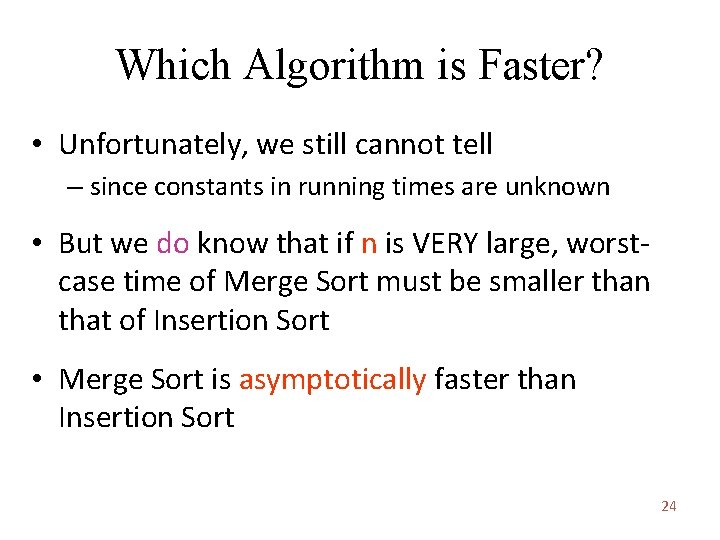Which Algorithm is Faster? • Unfortunately, we still cannot tell – since constants in running times are unknown • But we do know that if n is VERY large, worstcase time of Merge Sort must be smaller than that of Insertion Sort • Merge Sort is asymptotically faster than Insertion Sort 24Exercises • Problem 1 -1*, 2 -4 • Exercises 2. 2 -2, 2. 2 -4, 2. 3 -7* 25